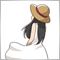# Indicator plots initially but not for any new candle!33

Hi all,

Thanks for looking at this thread. I'm looking to develop a custom indicator and it appears to be printing out the correct value initially but it just stops when a new candle appears! I don't know why and this is giving me a real headache!!!

Thanks for your help :)

```//+------------------------------------------------------------------+
//|                                        Momentum_RSI.mq5 |
//|                        Copyright 2018, MetaQuotes Software Corp. |
//|                                             https://www.mql5.com |
//+------------------------------------------------------------------+
#property copyright "Copyright 2018, MetaQuotes Software Corp."
#property version   "1.00"
//--- indicator settings
#property indicator_chart_window
#property indicator_buffers 4
#property indicator_plots 2

#property indicator_type1 DRAW_LINE
#property indicator_style1 STYLE_SOLID
#property indicator_width1 1
#property indicator_color1 0xFFAA00
#property indicator_label1 "Upper RSI Bound"

#property indicator_type2 DRAW_LINE
#property indicator_style2 STYLE_SOLID
#property indicator_width2 1
#property indicator_color2 0x0000FF
#property indicator_label2 "Lower RSI Bound"

//--- input parameters
input int InpPeriodRSI=14; // Period
input double InpRSIMultiplier=1.1; // Multiplier
//--- indicator buffers
double    UpperRSIBound[];
double    LowerRSIBound[];

//--- Value store arraus
double UpperRSIVal[];
double LowerRSIVal[];
double Close_prices[];

int ExtPeriodRSI;
//+------------------------------------------------------------------+
//| Custom indicator initialization function                         |
//+------------------------------------------------------------------+
int OnInit()
{
SetIndexBuffer(0,UpperRSIBound,INDICATOR_DATA);
PlotIndexSetDouble(0,PLOT_EMPTY_VALUE,0);
SetIndexBuffer(1,LowerRSIBound,INDICATOR_DATA);
PlotIndexSetDouble(1,PLOT_EMPTY_VALUE,0);
SetIndexBuffer(2,UpperRSIVal,INDICATOR_CALCULATIONS);
SetIndexBuffer(3,LowerRSIVal,INDICATOR_CALCULATIONS);
//--- indicator buffers mapping
//--- check for input
if(InpPeriodRSI<1)
{
ExtPeriodRSI=14;
Print("Incorrect value for input variable InpPeriodRSI =",InpPeriodRSI,
"Indicator will use value =",ExtPeriodRSI,"for calculations.");
}
else ExtPeriodRSI=InpPeriodRSI;
//---
return(INIT_SUCCEEDED);
}
//+------------------------------------------------------------------+
//| Custom indicator iteration function                              |
//+------------------------------------------------------------------+
int OnCalculate(const int rates_total,
const int prev_calculated,
const datetime &time[],
const double &open[],
const double &high[],
const double &low[],
const double &close[],
const long &tick_volume[],
const long &volume[],
{
//---
int    i,j;
double diff;

if(CopyClose(Symbol(), PERIOD_CURRENT, 0, rates_total, Close_prices) <= 0) return(rates_total);
ArraySetAsSeries(Close_prices, true);
//--- check for rates count
if(rates_total<=ExtPeriodRSI)
return(0);
//--- preliminary calculations
int pos=prev_calculated-1;
if(pos<=ExtPeriodRSI)
{
//--- first RSIPeriod values of the indicator are not calculated
UpperRSIVal=0.0;
LowerRSIVal=0.0;

double SumP=0.0;
double SumN=0.0;
for(i=1;i<=ExtPeriodRSI;i++)
{
UpperRSIVal[i]=0.0;
LowerRSIVal[i]=0.0;

diff=close[i]-close[i-1];
SumP+=(diff>0?diff:0);
SumN+=(diff<0?-diff:0);
}
//--- calculate first visible value
UpperRSIVal[ExtPeriodRSI]=SumP/ExtPeriodRSI;
LowerRSIVal[ExtPeriodRSI]=SumN/ExtPeriodRSI;
//--- prepare the position value for main calculation
pos=ExtPeriodRSI+1;
}

for(i=pos;i<rates_total-(ExtPeriodRSI+1) && !IsStopped();i++)
{
double SumPos=0.0;
double SumNeg=0.0;
for(j=i;j<i+ExtPeriodRSI;j++)
{
diff=close[j]-close[j-1];

SumPos+=(diff>0?diff:0);
SumNeg+=(diff<0?-diff:0);
}
UpperRSIVal[i]=SumPos/ExtPeriodRSI;
LowerRSIVal[i]=SumNeg/ExtPeriodRSI;

UpperRSIBound[i] = Close_prices[i]+UpperRSIVal[i]*InpRSIMultiplier;
LowerRSIBound[i] = Close_prices[i]-LowerRSIVal[i]*InpRSIMultiplier;
}

//--- return value of prev_calculated for next call
return(rates_total);
}
//+------------------------------------------------------------------+
```390

```if(CopyClose(Symbol(), PERIOD_CURRENT, 0, rates_total, Close_prices) <= 0) return(rates_total);
//ArraySetAsSeries(Close_prices, true);```
```for(i=pos;i<rates_total && !IsStopped();i++)
{
double SumPos=0.0;
double SumNeg=0.0;
for(j=i;j>i-ExtPeriodRSI;j--)```33